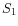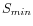## The algorithm

Consider an RNA molecule and one of its possible structures. In a stable solution there will be an equilibrium between unstructured RNA strands and RNA strands folded into. The propensity of a strand to leave a structure such as(the stability of), is determined by the free energy change involved in its formation. The structure with the lowest free energy () is the most stable and will also be the most represented structure at equilibrium. The objective of minimum free energy (MFE) folding is therefore to identifyamongst all possible structures.

In the following, we only consider structures without pseudoknots, i.e. structures that do not contain any non-nested base pairs.

Under this assumption, a sequence can be folded into a single coherent structure or several sequential structures that are joined by unstructured regions. Each of these structures is a union of well described structure elements (see below for a description of these). The free energy for a given structure is calculated by an additive nearest neighbor model. Additive, means that the total free energy of a secondary structure is the sum of the free energies of its individual structural elements. Nearest neighbor, means that the free energy of each structure element depends only on the residues it contains and on the most adjacent Watson-Crick base pairs.

The simplest method to identifywould be to explicitly generate all possible structures, but it can be shown that the number of possible structures for a sequence grows exponentially with the sequence length [Zuker and Sankoff, 1984] leaving this approach unfeasible. Fortunately, a two step algorithm can be constructed which implicitly surveys all possible structures without explicitly generating the structures [Zuker and Stiegler, 1981]: The first step determines the free energy for each possible sequence fragment starting with the shortest fragments. Here, the lowest free energy for longer fragments can be expediently calculated from the free energies of the smaller sub-sequences they contain. When this process reaches the longest fragment, i.e., the complete sequence, the MFE of the entire molecule is known. The second step is called traceback, and uses all the free energies computed in the first step to determine- the exact structure associated with the MFE. Acceptable calculation speed is achieved by using dynamic programming where sub-sequence results are saved to avoid recalculation. However, this comes at the price of a higher requirement for computer memory.

The structure element energies that are used in the recursions of these two steps, are derived from empirical calorimetric experiments performed on small molecules see e.g. [Mathews et al., 1999].

Subsections